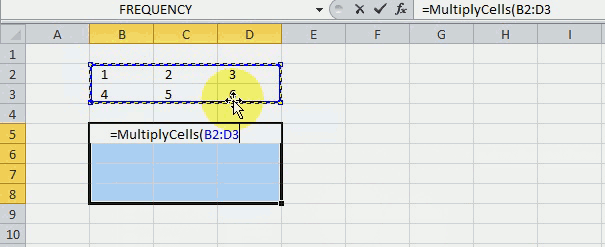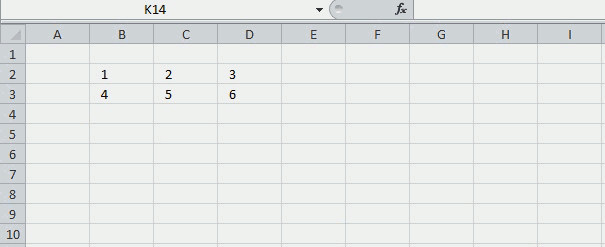Author: Oscar Cronquist Article last updated on December 31, 2018Hello Oscar,I have an Excel dataset consisting of 500 rows by 7 columns. I need to generate additional datapoints from this dataset. I want to multiply (or other function) each row by all 500 rows, creating 250,000 new rows of data. Each cell needs to function as a constant that is multiplied by all the other cells in the same column (which are not acting as constants).How do I do this efficiently? Thanks in advance!

I don't think you can multiply cells like that with an array formula, as far as I know.

Here is a user defined function I made, it multiplies each row by all other rows in the specified range.The picture above shows you the udf using values in cell range B2:B3 and returns the calculated values to cell range B5:D8.

### Function and argument

This is a user defined function or custom function, you need to copy code to code module before you can use it.

=MultiplyCells(range)

There is only one argument in this udf.

range - Cell range

### How do I use this?

Copy the following code to a module. How to insert a module to a workbook.

```Function MultiplyCells(rng As Range)
Dim rng1 As Variant
Dim tbl() As Variant
Dim rr As Single, r As Single, c As Single, tr As Single
rng1 = rng.Value
ReDim tbl(1 To rng.Cells.Rows.CountLarge ^ 2, 1 To rng.Cells.Columns.CountLarge)
tr = 1
For rr = LBound(rng1, 1) To UBound(rng1, 1)
For r = LBound(rng1, 1) To UBound(rng1, 1)
For c = LBound(rng1, 2) To UBound(rng1, 2)
tbl(tr, c) = rng1(rr, c) * rng1(r, c)
Next c
tr = tr + 1
Next r
Next rr
MultiplyCells = tbl
End Function

```

Enter this udf as an array formula, if the range has 2 rows and 3 columns enter the udf in a cell range with 4 (2*2) rows and 3 columns.

Here are the steps to enter this udf as an array formula

1. Select cell range
2. Type udf name and argument (=MultiplyCells(B2:D3)
3. Press and hold CTRL + SHIFT simultaneously
4. Press Enter
5. Release all keys

If you did it right the formula now has a curly bracket before and after. Like this {=MultiplyCells(B2:D3)}. Don't enter these yourself. If you are unsure, watch the formula bar carefully in the animated picture above.

Don't forget to save your workbook as a *.xlsm file.

### Learn code statements

The code explained here is located in the code module. You can find the code module in the VB Editor, press Alt+F11 to open the VB Editor. Click "Module" found in project explorer.

Function name and arguments

A user defined function always starts with "Function" and then a name. This udf has only one argument. Variable rng is a range object, read more about Defining data types.

`Function` `MultiplyCells(rng ``As` `Range)`

Create Custom Functions

Declaring variables

tbl() is an array and a variant variable, tbl has two parenthesis meaning it is an array. rng1  is also a variant.  rr, r, c and tr are Single variables. Read more about Defining data types.

```Dim rng1 As Variant
Dim tbl() As Variant
Dim rr As Single, r As Single, c As Single, tr As Single```

Dim statement

Save values from rng (range object) to rng1 (variant)

This speeds up the function considerably if you are working with large cell ranges. Excel copies all the values from the sheet and puts them in memory (array).

rng1 = rng.Value

Build array

ReDim dimensions the tbl array variant, it has the number of rows of the range argument with the power of 2. The values in the array are numbered from 1 to n. There are as many columns as in the range argument.

`ReDim` `tbl(1 ``To` `rng.Cells.Rows.CountLarge ^ 2, 1 ``To` `rng.Cells.Columns.CountLarge)`

ReDim Statement

Use variables r and c to save values in array

Variable tr keeps track of where to save the next column values in tbl.

tr = 1

For ... Next statement

Repeats a group of statements a specified number of times, here we want to multiply each row by all rows.

```For rr = LBound(rng1, 1) To UBound(rng1, 1)
For r = LBound(rng1, 1) To UBound(rng1, 1)
For c = LBound(rng1, 2) To UBound(rng1, 2)
```

For ... Next statement

Save value to tbl array

The variables tr, c, rr and r help us keep track of which values to use and where to save.

```tbl(tr, c) = rng1(rr, c) * rng1(r, c)
```

`tr = tr + 1`

Return tbl values to function

`MultiplyCells = tbl`

End udf

A function procedure ends with this statement.

`End Function`